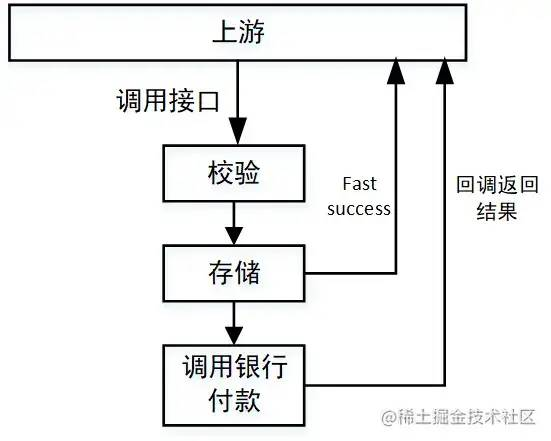##MSIPO技术圈 首页 IT技术 查看内容

# 接口性能优化技巧

2023-07-13• 数据库慢查询

• 业务逻辑复杂

• 线程池设计不合理

• 锁设计不合理

• 机器问题（fullGC，机器重启，线程打满）

• 万金油解决方式

| 慢查询（基于 mysql）

### ①深度分页

``````select name,code from student limit 100,20
```

```

``````select name,code from student limit 1000000,20
```

```

``````select name,code from student where id>1000000  limit 20
```

```

### ②未加索引

``````show create table xxxx（表名）
```

```

### ③索引失效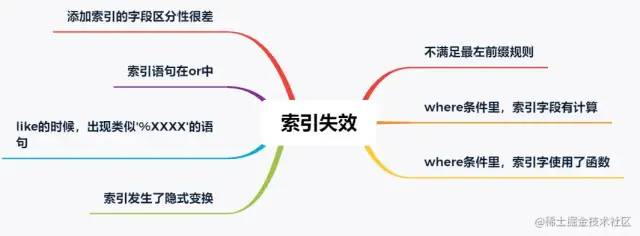• 某个字段只可能有 3 个值，那这个字段的索引区分度就很低。

• 再比如，某个字段大量为空，只有少量有值；

• 再比如，某个字段值非常集中，90% 都是 1，剩下 10% 可能是 2,3,4....

``````select name,code from student force index(XXXXXX) where name = '天才'
```

```

```其中 xxxx 是索引名。
```

### ⑤in 的元素过多

``````select id from student where id in (1,2,3 ...... 1000) limit 200
```

```

```

if (ids.

size()

>

200) {

throw new

Exception(

"单次查询数据量不能超过200");

}

```

| 业务逻辑复杂

### ①循环调用

```

List

<Model

> list

= new ArrayList

<>();

for(int i

=

0 ; i

<

12 ; i

+

+) {

Model model

= calOneMonthData(i);

/

/ 计算某个月的数据，逻辑比较复杂，难以批量计算，效率也无法很高

list.

}

```

```

/

/ 建立一个线程池，注意要放在外面，不要每次执行代码就建立一个，具体线程池的使用就不展开了

5,

5,

300L,

<>(

/

/ 开始多线程调用

List

<Future

<Model

>> futures = new ArrayList<>();

for(int i

=

0 ; i

<

12 ; i

+

+) {

Future

<Model

> future

> calOneMonthData(i););

futures.

}

/

/ 获取结果

List

<Model

> list

= new ArrayList

<>();

try {

for (int i

=

0 ; i

< futures.

size() ; i

+

+) {

list.

get(i).

get());

}

} catch (

Exception e) {

LOGGER.

error(

"出现错误：", e);

}

```

### ②顺序调用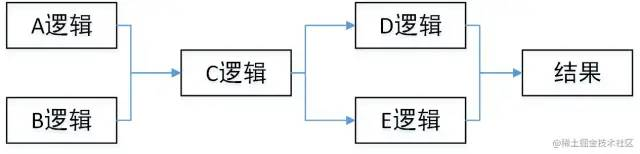```

A a

= do

A();

B b

= doB();

C c

= doC(a, b);

D d

= doD(c);

E e

= doE(c);

return doResult(d, e);

```

```

CompletableFuture

<A

> futureA

= CompletableFuture.supplyAsync(() -

> do

A());

CompletableFuture

<B

> futureB

= CompletableFuture.supplyAsync(() -

> doB());

CompletableFuture.allOf(futureA,futureB)

/

/ 等a b 两个任务都执行完成

C c

= doC(futureA.join(), futureB.join());

CompletableFuture

<D

> futureD

= CompletableFuture.supplyAsync(() -

> doD(c));

CompletableFuture

<E

> futureE

= CompletableFuture.supplyAsync(() -

> doE(c));

CompletableFuture.allOf(futureD,futureE)

/

/ 等d e两个任务都执行完成

return doResult(futureD.join(),futureE.join());

```

| 线程池设计不合理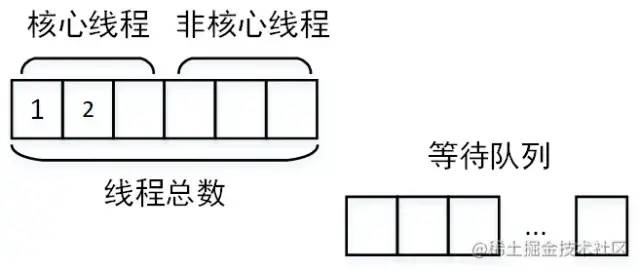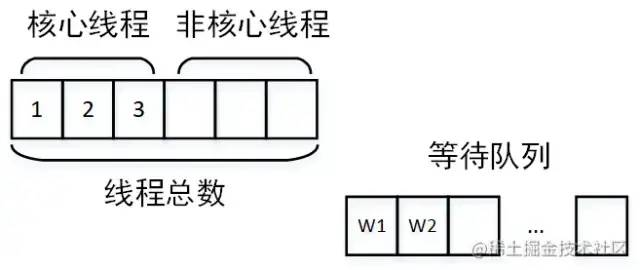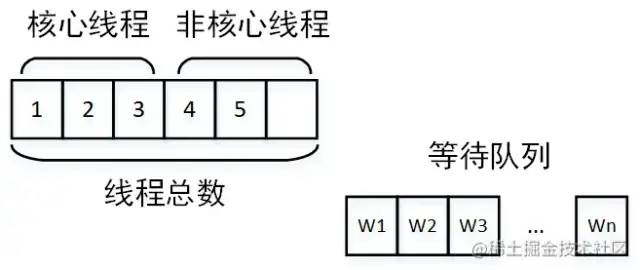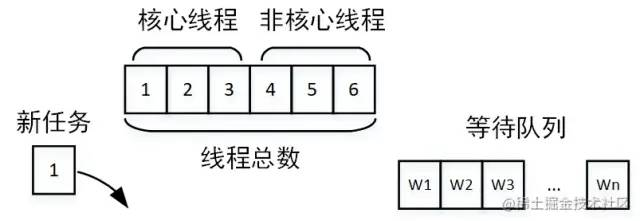• 核心线程设置过小：核心线程设置过小则没有达到并行的效果

• 线程池公用，别的业务的任务执行时间太长，占用了核心线程，另一个业务的任务到达就直接进入了等待队列

• 任务太多，以至于占满了线程池，大量任务在队列中等待

| 锁设计不合理

```

public

synchronized

void

doSome

() {

File

f

= calData();

sendSuccessMessage();

}

```

```

public

void

doSome(

) {

File f =

null;

synchronized(

this) {

f =

calData();

}

sendSuccessMessage();

}

```

| 机器问题（fullGC，机器重启，线程打满）

## 需要结合各种监控和具体场景具体分析，进而进行大事务拆分、重新规划线程池等等工作。

| 万金油解决方式

### ①缓存

• 简单的 map

• guava 等本地缓存工具包

• 缓存中间件：redis、tair 或 memcached

### ②回调 or 反查